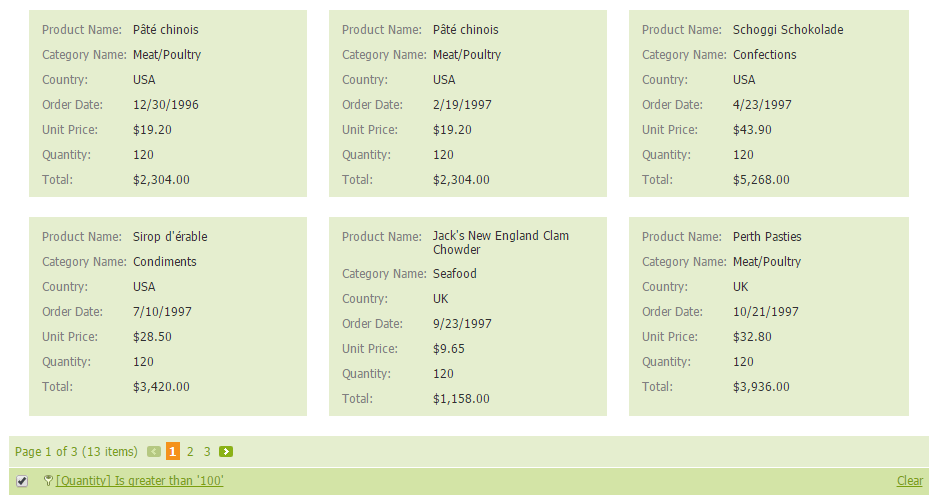19.2
19.1
18.2
18.1
17.2

## End-User Documentation

Assume that ASPxCardView is bound to a data table that contains the "UnitPrice" and "Quantity" fields. There is no field that represents the total sum, as this can be calculated manually as follows: UnitPrice*Quantity. This example shows how to add an unbound column to the ASPxCardView control to represent the total sum of an order.

The image below shows the result.``````    protected void CardView_Init(object sender, EventArgs e)
{
CardViewTextColumn colTotal = new CardViewTextColumn();
colTotal.Caption = "Total";
colTotal.FieldName = "Total";
colTotal.UnboundType = DevExpress.Data.UnboundColumnType.Integer;
colTotal.VisibleIndex = CardView.Columns.Count;
colTotal.PropertiesTextEdit.DisplayFormatString = "c2";